LR  >  Inequalities - Examples (with Solutions), Miscellaneous, Quantitative Reasoning

# Inequalities - Examples (with Solutions), Miscellaneous, Quantitative Reasoning - Notes | Study Reasoning Aptitude for Competitive Examinations - LR

 1 Crore+ students have signed up on EduRev. Have you?

Introduction

The expressions like 3x + 1 = x − 3 and x2 − 3x = 0 are equations. But which type of expressions is called
"inequations". When a statement written is neither true nor false until and unless it is replaced by some
numeral value, is called an open sentence. E.g. 7x + 5 > 19 is such that no conclusion can be made from this
unless x is replaced by some numeral values.

Now we can define inequalities as “a sentence which says that one thing is not equal to another. The two
sides are joined together by one of the following:
> (greater than);
< (less than);
≥ (greater than or equal to)
≤ (less than or equal to)

Example: 3x + 1 > x − 3 or x2 − 3x ≤ 0
As is the case with equations, they are ordered by degree and by the number of unknowns.
In the above two examples, the first will be a linear inequality with one unknown and the second will be

The questions related to inequalities are mainly of two types i.e.

• Direct Inequalities
• Indirect Inequalities

The detailed explanations for both these types are given below along with several solved examples to help the CAT aspirants understand the topic in a better way.

Some Specific Rules to Solve Inequalities:

• A relation can be defined only if the statements of inequalities have a common term. For example, if A > B and B < C, it can be concluded that A > C.
• A relation is considered undefined if the inequalities do not have a common term. For example, if A > B and C > D, the relation between A and D is undefined.
• In case of complementary pairs, only the complementary relation is defined. For example, if A ≥ B and B ≥ C, the relation between A and C can only be A ≥ C and not A > C.

Direct Inequalities:

The questions related to direct inequalities only includes basic symbols of inequalities like <, >, =, ≥, etc. In these questions, the candidates are given a set of sentences followed by a set of conclusions. The candidates are required to analyze the conclusion and answer the question accordingly.

Examples of Direct Inequality Reasoning Questions :

Few sentences are given along with two conclusions. Read the statements and check which conclusion is true.

Direct Inequality Question- 1:

Statements:

1. A > B = C
2. G < C > D

Conclusions:

1. A < D
2. A = G

(a) Only conclusion I holds true.
(b) Only conclusion II holds true.
(c) Either conclusion I or II holds true.
(d) Neither conclusion I nor II holds true.
(e) Both conclusions I and II hold true.

Solution:

Option (d)

Reason:

From the given statements, it can be concluded that A must be greater than D as D < C < A.

Also, as C > G, A can never be equal to G.

Direct Inequality Question- 2:

Statement:

1. I ≥ U > T ≤ R
2. U ≥ V = W > C

Conclusions:

1. W > T
2. C > I

(a) Only conclusion I holds true.
(b) Only conclusion II holds true.
(c) Either conclusion I or II holds true.
(d) Neither conclusion I nor II holds true.
(e) Both conclusions I and II hold true.

Solution:

Option (d)

Conclusion 1 does not holds true as T < U while U ≥ W. Similarly, conclusion 2 also does not hold since I ≥ U and U ≥ C.

Coded Inequalities:

The questions related to coded inequalities involve codes like @, #, &, etc. to define a specific relation between different entities. So, in these type of questions, the candidates need to analyze (or write down) the coded relations in standard form and then check the conclusions to answer the question. They are also called symbol operations and are repeated often in CAT exams.

Examples of Coded Inequality Reasoning Questions :

Few sentences are given along with two conclusions. Read the statements and check which conclusion is true.

Coded Inequality Question- 1:

In the given statements, the relations are represented as follows:

P # Q‘ means ‘P is not smaller than Q’.

P \$ Q‘ means ‘P is neither smaller than nor equal to Q’.

P % Q‘ means ‘P is neither greater than nor smaller than Q’.

P ^ Q’ means ‘P is not greater than Q’.

P & Q’ means ‘P is neither greater than nor equal to Q’.

Statements:

1. X # Y
2. Y \$ Z
3. A & Z

Conclusions:

1. Z & X
2. A \$ Y

(a) Only conclusion I holds true.
(b) Only conclusion II holds true.
(c) Either conclusion I or II holds true.
(d) Neither conclusion I nor II holds true.
(e) Both conclusions I and II hold true.

Solution:

Option (a)
At first, the code must be interpreted in the general form. Here the symbols can be interpreted as :

# means

\$ means > and

& means <

Representing the statements in general form gives:

• X ≥ Y
• Y > Z
• A < Z

Similarly, the conclusions can be interpreted as, Z < X and A > Y.

Thus, from the given conclusions, Z < X holds true. But as A < Z and Z < Y, the conclusion A > Y is false. So, only the first conclusion follows.

Solving Inequation
On the basis of the laws of inequality, we have the following working rules.

1. Rule of Addition and Subtraction: Adding or subtracting a fixed number to each side of an inequality
produces an equivalent inequality.
Example: Adding 2 to each side of the inequality x – 2 ≤ 1 is equivalent to x ≤ 3.

2. Rule of multiplication/division by a positive number: All terms on both sides of an inequality can be
multiplied or divided by a positive number.

3. Rule of multiplication/division by a negative number: If all terms on both sides of an inequality are
multiplied or divided by a negative number, the sign of the inequality will be reversed.
Example: If 6x – 4 < – 8x + 6 then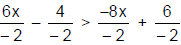or – 3x + 2 > 4x – 3

Using the above facts we take the following steps to solve linear inequation:
ax + b > cx + d.

Step 1: Make all terms containing the variable (unknown) x positive and on one side of the inequation,
and the constants on the other side by using rule 1.

Step 2: Put the inequation in the form px > q or px < q

Step 3: Divide both sides by positive p, using rule 3.
Inequalities
Graphing Solutions of Inequalities

Representation of the real number set R and its subsets:

The solution of a linear inequation in one variable consists of many real numbers but may not all real
numbers. As such the set of solutions is always a subset of real numbers.

Emphasize the fact that solving an inequality means describing a set not just finding a number. This set is the
solution set of the problem. To visualize the solution set, draw it on the number line. To do this, draw the
number line and shade the part of the number line corresponding to the solution set. The solution set to a
single inequality will be a half line that may or may not include the endpoint. When the endpoint is included,
draw a solid dot for the endpoint. When the endpoint is not included, draw a circle.

Ex.1 Represent the solution set of x ≤ 5 on a number line.
Sol. The solution set of x ≤ 5 is represented by the set of point to the left of 5 on the number line. It does
include the endpoint, since x = 5 is included.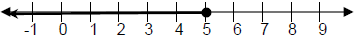Solution Sets
The solution of any inequation is always expressed in a solution set. These solution the basis of requirement
of the elements contained in them can be classified as below:

a. Ranges where the ends are excluded: If the value of x is denoted as (1, 2) it means 1 < x < 2 i.e. x is
greater than 1 but smaller than 2.

b. Ranges where the ends are included
[2, 5] means 2 ≤ x ≤ 5

c. Mixed Ranges
(3, 21] means 3 < x ≤ 21

d. If solution set = {1, 2} means x = 1 and 2 only.

Ex.2 Solve for x, – 2x > 4

Sol. – 2x > 4
Divide both sides by – 2,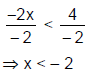Graphically, the solution is: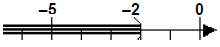Some more examples: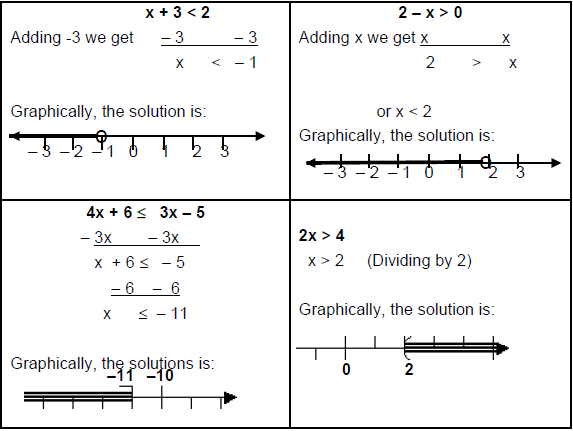Ex.3 P is the solution set of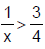and R is the solution set of : 5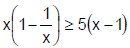. Where x ∈ W, find
the set P ∩ R.

Sol. For finding the solution set of P solve the inequation.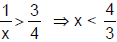i.e. x < 1.33, but x ∈ W.
So solution set of P = {1} since for x = 0 the inequation is not defined
Now for solution set of R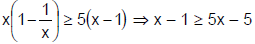when x ≠ 0.

Solving we have, x ≤ 1 but x ≠ 0. Hence solution set R = {1}
P ∩ R = {1}.

Properties of inequalities

(i) If a > b and b > c, then a > c.

(ii) If a > b and c is any real number, then a + c > b + c and a – c > b – c.

(iii) If a1 > b1, a2, > b1, ….an > bn, then a1 + a2 +…….an > b1 + b2 + ….bn.

(iv) If a1 > b1, a2 > b2, ……..an > bn and the a’s and the b’s are all positive real numbers. Then a1 . a2….an >
b1 . b2….bn.

(v) If a > b > 0, then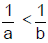(vi) If a > b > 0 and n is a positive integer,
then an > bn, a–n < b-n and a1/n > b1/n provided in the last inequality only real
positive values of the nth roots are taken into account.

 Do you know?If a1 > b1 and a2 > b2, then it does not necessarily follow that a1 - a2 > b1 - b2

(vii) If x > y > 0, then loga x > loga y if a > 1and loga x < loga y if 0 < a < 1.

(viii) If x > y > 0, then ax > ay if a > 1, and ax < ay if 0 < a < 1.

(ix) If a > b > 0 and x > 0, then ax > bx.

(x) The Arithmetic mean of n positive numbers is always greater than their Geometric means.
i.e. if a1, a2. …. an are all positive then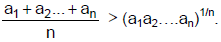(xi) If the sum of n positive numbers is a constant, then their product is
maximum when they are all equal; if their product is a constant their sum is
minimum when they are equal.

i.e. If a1 + a2 + …..+ an = constant k, where a’s are all positive, then a1 .
a2…an is maximum when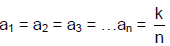Also, if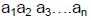,= constant k where the a’s are all positive, then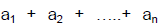is Minimum
when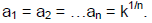CHALLENGER

list one of the solution set of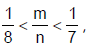where m, n ∈ Z

(xii) a2 + b2 + c2 > ab + be + ca
(xiii) (n!)2 > nn , for all n > 2

(xiv)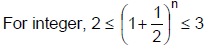(xv) a2b + b2c + c2a > 3abc

(xvi)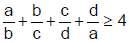(xvii)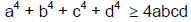Ex.4 What values for X satisfy the inequality x2 – 10x + 21 <0
(1) x < 3

(2) x > 7

(3) 3 < x < 7

(4) No solution.
Sol. x2 −10x + 21< 0 means (x – 3) (x – 7) < 0. So x – 3 < 0 and x – 7 > 0 or x – 3 > 0 and
x – 7 < 0. By drawing on the number line, the intersecting part will be 3 < x < 7.

(or)

Go with the options.
It is very simple to solve the inequality questions from the options.
Take option (1), since it is x<3, take any value for x, which is less than 3. If the inequality does not
satisfy, then we can eliminate this option. Some times two options satisfy the inequality. Then we have
to pick the option which has maximum range.

Here x = 2 does not satisfy the inequality, hence option (1) is eliminated.
Similarly x = 8 does not satisfy. So option (2) also can be eliminated.
Since the inequality is satisfied for x = 4, 5,… Option (3) is the required answer.

Ex.5 If x3 – 3x2 – 6 x + 8 > 0; then X can be best described by,
(1) x < 4

(2) – 2 < x < 1

(3) x > 4

(4) both (2) and (3)

Sol. It is better to solve this with the help of options

x = 3 does not satisfy the inequality, so option (1) is eliminated.

The inequality is satisfied by all the values of option (2) and the values of option (3) also satisfy this. So

Solving linear inequalities, such as "x + 3 > 0", was pretty straightforward, as long as you remembered to flip
the inequality sign whenever you multiplied or divided throughout by a negative number (as you would when
solving something like "– 2x < 4").

We can follow the same method of finding intercepts and using graphs to solve inequalities containing
quadratics. When we have an inequality with "x2" as the highest-degree term, it is called a "quadratic
inequality".

Let us consider a quadratic equation having two roots a and b, such that a > b. then
(i) (x – a) (x – b) > 0
(ii) (x – a) (x – b) < 0 are the possible inequalities.
If we plot the graph of the quadratic equation with roots a and b, we get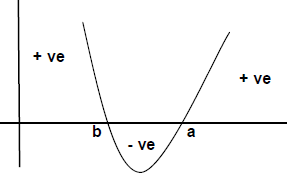So from the graph the solution of the above two equations are
(i) Either x < b or x > a
(ii) But on the other end the value b < x < a is the solution of equation 2.

Ex.6 – x2 + 4 < 0.
Sol. – x2 + 4 < 0.
4 – x2 < 0.
(2 – x) (2 + x) < 0.

CASE 1.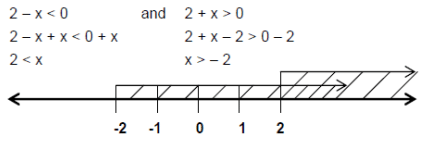From the number line we have
x > 2 ……..(1)

CASE 2.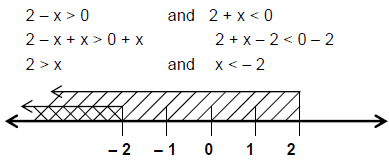Image caption

So the solution set x < – 2
Solution Set = {x > 2 or x < – 2}

Higher Degree Inequations

The polynomial inequations of degree 3 or more are polynomials of higher degree. Let us consider an
inequation of degree 3.
(x + 1) (x – 2) (x – 4) > 0 ………….. (1)

Well this is the inequation of as the multiplication of three numbers whose result should be positive i.e. p x q x
r > 0 where p, q & r are real numbers. The product of three real numbers should be positive if all of them are
positive or at least two of them positive.

In the given equation we have three points where the inequation change its nature. These points are x = (– 1,
2, 4). The nature of inequation can be explained below in the table –

 Value of x (x + 1) (x - 2) (x - 4) (x + 1) (x - 2) (x - 4) x < -1 - ve -ve -ve (x+ 1)(x -2 )(x-4)< 0 -1< x < 2 + ve -ve -ve (x + 1)(x - 2) (x - 4)>0 2< x < 4 + ve + ve -ve (x + 1) (x - 2) (x - 4) < 0 x > 4 + ve + ve + ve (x + 1) (x - 2) (x - 4) > 0

So from the table we have the solution set of the inequation.

Solution Set = – 1 < x < 2 or x > 4

If we wish to solve the above inequation by graphical method then the graph will be as follows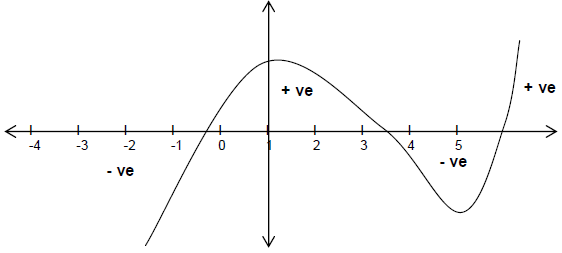Ex.7 Solve the inequation graphically and give the general solution of both the equations.
i. (X – a) (X – b) (X – c) (X – d) > 0
ii. (X – a) (X – b) (X – c) (X – d) < 0,
Where a, b, c & d are real numbers and a < b < c < d

Absolute value
The absolute value |x|, read as the modulus of x of a real number x is defined as
|x| = x if x ≥ 0 and |x| = – x, if x < 0.
Thus |x| ≥ 0; |x| = |– x|

Modulus and inequality
General solution of the inequation containing modulus:

(a) If |x| = c ⇒ x = {– c, c}
(b) If |x| > c ⇒ x > c or x < – c
(c) If x < c ⇒ – c < x < c.

Ex.8 What is the solution set of equation x2 + 5|x| + 6 > 0 ?

Sol. Case 1: If x ≥ 0, then |x| = x
So we have,    x2 + 5x + 6 > 0
⇒ (x + 2) (x + 3) > 0
When both positive we get, x > -2 but x ≥ 0
Hence solution set = {x | x ≥ 0 and x ∈ R} …………(1)
When both negatives then we have, x < – 3 but x is positive, So no solution.

Case 2: If x < 0, then |x| = -x
We have, x2 – 5x + 6 > 0
⇒ (x – 2) (x – 3) > 0
Solving the inequality we will have x > 3 or x < 2 but here x < 0
So solution set = {x | x < 0 & x ∈ R} ……………(2)
From equation 1 and 2 we have x ∈ R is the solution of the given equation.

 Toolkit(i) |x - a| = x - a if x > a and |x - a| = a - x, if x < a(ii)  If b > 0, then |x - a| 0, then |x|< b ⇒ -b < x < b(iii) |x - a|>b ⇒ x - a > b or a - x > b ⇒ x > a + b or x < a - b(iv) |x - a| = b ⇒ x - a = b or a - x = b ⇒ x = a + b or x = a - b(v) |a + b| < |a| + |b|, i.e (sum) < sum of moduli(vi) |a - b| > |a| - |b|(vii)  |a b| = |a| |b|, mod (product) = product of moduli

Ex.9 Solve |2x + 3| < 6.

Sol. Since this is a "less than" absolute value inequality, the first step is to clear the absolute value
according to the pattern:
|2x + 3| < 6
– 6 < 2x + 3 < 6 [this is the pattern for "less than"]
– 6 – 3 < 2x + 3 – 3 < 6 – 3
– 9 < 2x < 3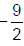< x <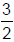Then the solution to |2x + 3| < 6 is the interval< x <Best approach to solve the inequality questions is to approach the questions by options.

Ex.10 Solution of the inequality: |x + 2| > |3x – 5| is
(1)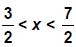(2)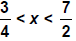(3)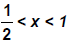(4)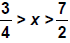(5)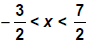Sol. Pick any option & check any value of x satisfying the inequality.
Let us take x = 2 which lies in option (1) & (2) & (5), If x = 2 satisfies the given equation then one out of
(1) or (2) or (5) is the answer otherwise one out of (3) or (4) is the answer.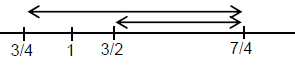Put x = 2 in |x + 2| > |3x – 5| we get
4 > 1 which is true so choice (3) & (4) can be eliminated

Put x = 1 we get
3 > 2 which is true and hence (1) is eliminated, because it doesn’t has x = 1.
Now take x = – 1, we get 1 > 8, which is wrong, so option (5) is eliminated.

The document Inequalities - Examples (with Solutions), Miscellaneous, Quantitative Reasoning - Notes | Study Reasoning Aptitude for Competitive Examinations - LR is a part of the LR Course Reasoning Aptitude for Competitive Examinations.
All you need of LR at this link: LR

## Reasoning Aptitude for Competitive Examinations

12 videos|20 docs|116 tests

## Reasoning Aptitude for Competitive Examinations

12 videos|20 docs|116 tests

Track your progress, build streaks, highlight & save important lessons and more!

,

,

,

,

,

,

,

,

,

,

,

,

,

,

,

,

,

,

,

,

,

,

,

,

,

,

,

;